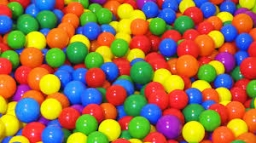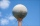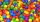# Thousand balls

We have to create a thousand balls from a sphere with a diameter of 1 m. What will be their radius?

r =  5 cm

### Step-by-step explanation:Did you find an error or inaccuracy? Feel free to write us. Thank you!Tips to related online calculators
Do you know the volume and unit volume, and want to convert volume units?

#### You need to know the following knowledge to solve this word math problem:

We encourage you to watch this tutorial video on this math problem:

## Related math problems and questions:

• Cube basicsHow long is the edge length of a cube with volume 15 m3?
• Volume per timeHow long does fill take for a pump with a volume flow of 200 l per minute fill a cube-shaped tank up to 75% of its height if the length of the cube edge is 4 m?
• The cubeThe cube has a surface area of 486 m ^ 2. Calculate its volume.
• Cube V2SThe volume of the cube is 27 dm cubic. Calculate the surface of the cube.
• EightEight small Christmas balls with a radius of 1 cm have the same volume as one large Christmas ball. What has a bigger surface: eight small balls, or one big ball?
• Volume of sphereHow many times does the volume of a sphere increase if its radius increases 2 ×?
• The cubeThe cube has a surface of 600 cm2. What is its volume?
• Iron sphereIron sphere has weight 100 kg and density ρ = 7600 kg/m3. Calculate the volume, surface, and diameter of the sphere.
• Plasticine ballPlasticine balls have radius r1=85 cm, r2=60 mm, r3=59 cm, r4=86 cm, r5=20 cm, r6=76 mm, r7=81 mm, r8=25 mm, r9=19 mm, r10=14 cm. For these balls
• Cube in a sphereThe cube is inscribed in a sphere with a volume 7253 cm3. Determine the length of the edges of a cube.
• The reservoirThe reservoir has the shape of a sphere with a diameter of 7.5 m. How many hectoliters can max fit in a reservoir?
• Hectoliter into cubeCan 1hl of water fit into a half-meter cube?
• The volumeThe volume of the rotating cone is 376.8cm³, the height of this cone is 1dm. Calculate the diameter of the cone base.
• Cylinder containerThe cylindrical container with a diameter of 1.8 m contains 2,000 liters of water. How high does the water reach?
• An exampleAn example is playfully for grade 6 from Math and I don't know how to explain it to my daughter when I don't want to use the calculator to calculate the cube root. Thus: A cuboid was made from a block of 16x18x48 mm of modeline. What will be the edge of t
• Clay ballsHow many clay balls with a radius of 1 cm can be made from a ball of clay with a radius of 8 cm?
• Water tank300hl of water was filled into the tank 12 m long and 6 m wide. How high does it reach?